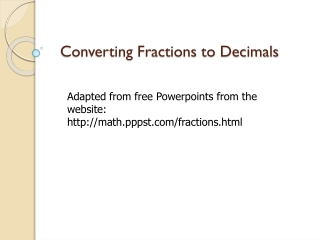DownloadDownload PresentationConverting Fractions to Decimals

# Converting Fractions to Decimals

Télécharger la présentation## Converting Fractions to Decimals

- - - - - - - - - - - - - - - - - - - - - - - - - - - E N D - - - - - - - - - - - - - - - - - - - - - - - - - - -
##### Presentation Transcript

1. Converting Fractions to Decimals Adapted from free Powerpoints from the website: http://math.pppst.com/fractions.html

2. Discussion • Explain what a fraction is • Explain what a decimal is • Find a decimal that is equivalent to ¼ • What method did you use to find this?

3. Problem of the Day Complete the following Compare 5/13 to 4/9 Compare 0.384 to 0.4

4. Fraction is a comparison of two numbers • 5/13 to 4/9 • To compare the size of each part, the “whole” must be cut into the same size; the denominators must be the same

5. Converting Fractions to Decimals • To convert a Fraction to a Decimal manually, follow these steps: • Step 1: Find a number you can multiply by the bottom of the fraction to make it 10, or 100, or 1000, or any 1 followed by 0s. • Step 2: Multiply both top and bottom by that number. • Step 3. Then write down just the top number, putting the decimal place in the correct spot (one space from the right for every zero in the bottom number)

6. Method #1: Converting with Proportions • Example # 1: Express 3/4 as a Decimal • Step 1: We can multiply 4 by 25 to become 100 • Step 2: Multiply top and bottom by 25 3 = 75 4 100

7. Example # 1 continued…. • Step 3: Write down 75 with the decimal place 2 spaces from the right (because 100 has 2 zeros); • Answer = 0.75 • Can you explain what we just did? • Try to express ¼ as a decimal using method # 1.

8. Method #2: Convert by Dividing • Example #1: to write 5/8 as a decimal, we need to calculate 5 ÷ 8: 0.625 8 √ 5.000 So = 0.625 as a decimal. • Try this example: write 4/5 as a decimal using division (method #2).

9. .80 4 0 0

10. Lets Practice!! • We are going to use this website to fish out some fractions and decimals. • Lets see how well you understand this lesson. • Go to www.iknowthat.com • Select “Math” in the left margin • Select “Fishy Fractions” • Select “Fractions and Decimal Match”